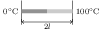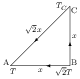# Heat Conduction in One Dimension

## Problems from IIT JEE

Problem (IIT JEE 2004): Two identical conducting rods are first connected independently to two vessels, one containing water at $100\;\mathrm{{}^{o}C}$ and the other containing ice at $0\;\mathrm{{}^{o}C}$. In the second case, the rods are joined end to end and connected to the same vessels. Let $q_1$ and $q_2$ grams per second be the rate of melting of ice in the two cases, respectively. The ratio $q_1/q_2$ is,

1. $1/2$
2. $2$
3. $4$
4. $1/4$

Solution: The rate of heat conduction through a material having conductivity $\kappa$, cross-section area $A$, length $\Delta x$, and temperature difference between two ends $\Delta T$ is given by, \begin{align} {\Delta Q}/{\Delta t}=\kappa A {\Delta T}/{\Delta x}.\nonumber \end{align}In case (1), two rods are connected in parallel. The rate of heat transfer through each rod is, ${\Delta Q}/{\Delta t}=\kappa A ({100}/{l})$. Thus, the rate of heat transfer to the ice is, \begin{align} \label{vpa:eqn:1} {\Delta Q_1}/{\Delta t}=2({\Delta Q}/{\Delta t})=\kappa A({200}/{l}). \end{align}In case (2), two identical rods are connected in series making their effective length $2l$. The rate of heat transfer to the ice is given by, \begin{align} \label{vpa:eqn:2} {\Delta Q_2}/{\Delta t}=\kappa A({100}/{2l}). \end{align} The heat transferred to the ice is used to melt it. The rate of melting is, \begin{align} q={\Delta m}/{\Delta t}=(1/L)\Delta Q/\Delta t, \nonumber \end{align} where $L$ is latent heat of fusion. Use above equations to get the ratio of rate of melting in two cases i.e., $q_1/q_2=\frac{\Delta Q_1}{\Delta t}/\frac{\Delta Q_2}{\Delta t}=4$.

Problem (IIT JEE 1995): Three rods of identical cross-sectional area and made from the same metal form the sides of an isosceles triangle ABC, right angled at B. The points A and B are maintained at temperatures $T$ and $\sqrt{2}T$, respectively. In the steady state, the temperature of the point C is $T_c$. Assuming that only heat conduction takes place, $T_c /T$ is,

1. $\frac{1}{2\left(\sqrt{2}-1\right)}$
2. $\frac{3}{\sqrt{2}+1}$
3. $\frac{1}{\sqrt{3}\left(\sqrt{2}-1\right)}$
4. $\frac{1}{\sqrt{2}+1}$

Solution:Let $T_A=T$, $T_B=\sqrt{2}T$, and the steady state temperature of C is $T_C$. Since $T_B > T_A$, heat flows from B to A. If $T_CC from A as well as from B leading to a non-steady state. Similarly, if$T_C>\sqrt{2}T$then heat flows from C to A as well as to B leading to a non-steady state. Thus, in steady state$TB to C is equal to heat flow from C to A (see figure). The rates of heat flow from B to C and from C to A are given by, \begin{align} \label{poa:eqn:1} &{\mathrm{d}Q_\text{BC}}/{\mathrm{d}t}={\kappa A(T_B-T_C)}/{x}={\kappa A(\sqrt{2}T-T_C)}/{x},\\ \label{poa:eqn:2} &{\mathrm{d}Q_\text{CA}}/{\mathrm{d}t}={\kappa A(T_C-T_A)}/{\sqrt{2}x}={\kappa A(T_C-T)}/{\sqrt{2}x}. \end{align} In steady state, ${\mathrm{d}Q_\text{BC}}/{\mathrm{d}t}={\mathrm{d}Q_\text{CA}}/{\mathrm{d}t}$. Use above equations to get, \begin{align} T_C={(T_A+\sqrt{2}T_B)}/{(1+\sqrt{2})}={3T}/{(1+\sqrt{2})}.\nonumber \end{align}

Problem (IIT JEE 1991): A point source of heat of power $P$ is placed at the centre of a spherical shell of mean radius $R$. The material of the shell has thermal conductivity $K$. If the temperature difference between the outer and inner surface of the shell is not to exceed $T$, the thickness of the shell should not be less than ______.

Solution The heat produced per unit time by the source is $P$. This heat is distributed over inner surface of the spherical shell having area $A=4\pi R^2$. Let $x$ be thickness of the shell. The rate of heat transfer due to conduction is, \begin{align} {\mathrm{d}Q}/{\mathrm{d}t}={K A T}/{x}, \end{align} ]where $T$ is temperature difference between inner and outer surfaces of the shell. For the temperature $T$ to be constant, the rate of heat incident on the inner surface of the shell should be equal to the rate of heat transfer through conduction i.e., $P={\mathrm{d}Q}/{\mathrm{d}t}$. Use above equation to get, \begin{align} x={KAT}/{P}={4\pi R^2 KT}/{P}.\nonumber \end{align}